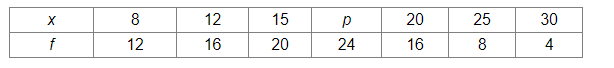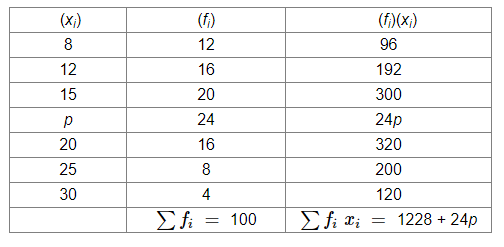# Find the value of p for the following frequency distribution whose mean is 16.6Question:

Find the value of p for the following frequency distribution whose mean is 16.6Solution:

We will make the following table:Thus, we have:

Mean $=\frac{\sum f_{i} x_{i}}{\sum x_{i}}$

$\Rightarrow 16.6=\frac{(1228+24 p)}{100}$

$\Rightarrow 1660=1228+24 p$

$\Rightarrow 24 p=432$

$\Rightarrow p=18$